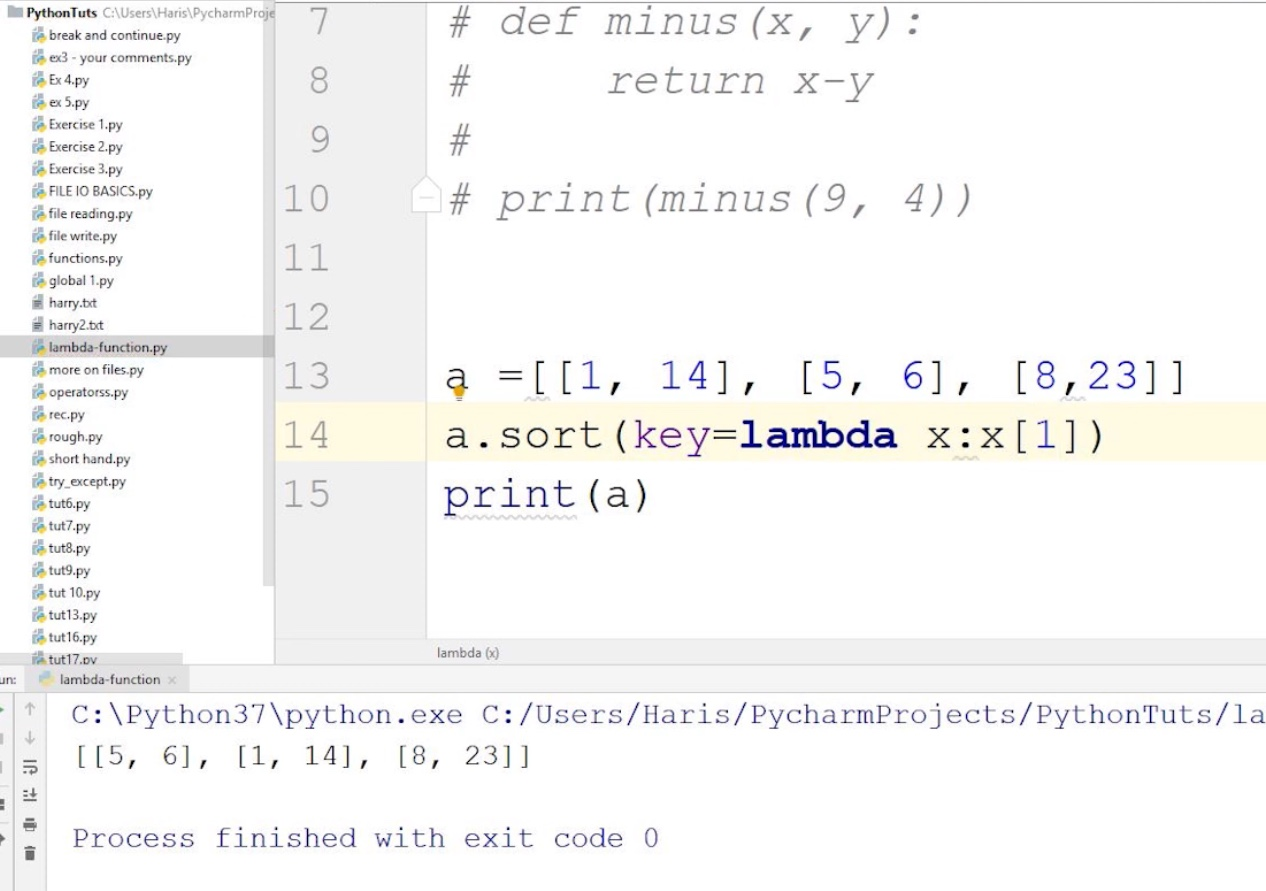### Map, Filter & Reduce

Python provides many built-in functions that are predefined and can be used by the programmers by just calling them. These functions not only ease our work but also create a standard coding environment. In this tutorial, we will learn about three important functions: map(), filter, and reduce() in Python. These functions are most commonly used with Lambda function. "Lambda functions are functions that do have any name". These functions are used as parameters to other functions. If you do not know what lambda functions are, then I recommend you to check Anonymous/Lambda Functions In Python  tutorial first to avoid any confusion.Figure 1:Anonymous/Lambda Functions In Python

#### Why are lambdas relevant to map(), filter() and reduce()?

These three methods expect a function object as the first argument. This function object can be a normal or predefined function. Most of the time, functions passed to map(), filter(), and reduce() are the ones we only use once, so there's often no point in defining a named function(def function).To avoid defining a new function, we write an anonymous function/lambda function that we will only use once and never again.

#### map():

"A map function executes certain instructions or functionality provided to it on every item of an iterable."

The iterable could be a list, tuple, set, etc. It is worth noting that the output in the case of the map is also an iterable i.e., a list. It is a built-in function, so no import statement required.

#### SYNTAX:

``map(function, iterable) ``

A map function takes two parameters:

• First one is the function through which we want to pass the items/values of the iterable
• The second one is the iterable itself

#### Example:

``````items = [1, 2, 3, 4, 5]
a=list(map((lambda x: x **3), items))
print(a)
#Output: [1, 8, 27, 64, 125]``````

The map()function passes each element in the list to a lambda function and returns the mapped object.

#### filter():-

"A filter function in Python tests a specific user-defined condition for a function and returns an iterable for the elements and values that satisfy the condition or, in other words, return true."

It is also a built-in function, so no need for an import statement. All the actions we perform using the filter can also be performed by using a for loop for iteration and if-else statements to check the conditions. We can also use a Boolean that could take note of true or false, but that would make the process very lengthy and complex. So, to simplify the code, we can use the filter function.

#### SYNTAX:

``filter(function, iterable)``

It also takes two parameters:

• First one is the function for which the condition should satisfy
• The second one is the iterable

#### Example:

``````a = [1,2,3,4,5,6]
b = [2,5,0,7,3]
c= list(filter(lambda x: x in a, b))
print(c) # prints out [2, 5, 3]``````

#### reduce():

"Reduce functions apply a function to every item of an iterable and gives back a single value as a resultant".

Unlike the previous two functions (Filter and Map), we have to import the reduce function from functools module using the statement:

from functools import reduce

We can also import the whole functools module by simply writing

Import functools

But in the case of bigger projects, it is not good practice to import a whole module because of time restraint.

#### SYNTAX:

``reduce(function, iterable)``

#### Example:

``````from functools import reduce
a=reduce( (lambda x, y: x * y), [1, 2, 3, 4] )
print(a)
#Output: 24``````

Like the previous two, it also takes two-parameter. First one is the function and the second one is the iterable

Its working is very interesting as it takes the first two elements of the iterable and performs the function on them and converts them into a single element. It proceeds further, taking another element and performing the function of that one and the new element. For example, if we have four digits and the function wants to multiply them, then we can first multiply the first two and then multiply the third one in their resultant and then the forth and so on. The reduce is in the functools in Python 3.0. It is more complex. It accepts an iterator to process, but it is not an iterator itself. It returns a single result.

#### Code file as described in the video

``````#--------------------------MAP------------------------------
# numbers = ["3", "34", "64"]
# numbers = list(map(int, numbers))

# for i in range(len(numbers)):
#     numbers[i] = int(numbers[i])

# numbers = numbers + 1
# print(numbers)

# def sq(a):
#     return a*a
#
# num = [2,3,5,6,76,3,3,2]
# square = list(map(sq, num))
# print(square)
# num = [2,3,5,6,76,3,3,2]
# square = list(map(lambda x: x*x, num))
# print(square)

# def square(a):
#     return a*a
#
# def cube(a):
#     return a*a*a

# func = [square, cube]
# num = [2,3,5,6,76,3,3,2]
# for i in range(5):
#     val = list(map(lambda x:x(i), func))
#     print(val)

#--------------------------FILTER------------------------------
# list_1 = [1,2,3,4,5,6,7,8,9]
#
# def is_greater_5(num):
#     return num>5
#
# gr_than_5 = list(filter(is_greater_5, list_1))
# print(gr_than_5)
#--------------------------REDUCE------------------------------
from functools import reduce

list1 = [1,2,3,4,2]
num = reduce(lambda x,y:x*y, list1)
# num = 0
# for i in list1:
#     num = num + i
print(num)

``````nazishali 7 months, 1 week ago
`Today I finished this video and I am also making my files in pycharm. I just want to know how to import file on GitHub like profile.`harry 4 months, 4 weeks ago
`ohh great. Watch my github tutorial for more!`sibu 5 months ago
`harry bhai yaha tak dekha hai'`harry 4 months, 4 weeks ago
`Great bro! I wish you all the best in learning python buddy!`p2499 1 month, 3 weeks ago
```Thank u soo much sir for the videos
```

#### Resources

No downloadable resources for this video. If you think you need anything, please post it in the QnA!

#### Course Announcements

Any Course related announcements will be posted here